JOINProblem Statement
Contest: 2010 TCO Marathon Final
Problem: CollapsingMaze

### Introduction

You are collecting coins in a maze underground. Luckily for you, you have a complete map of the maze that shows the locations of all the coins. Unluckily, however, there has just been an earthquake and the maze is extremely unstable. Sections of the maze are collapsing all the time. But, do not despair because you also have drilling equipment which will allow you to go through the rock (including clearing out collapsed sections).

### Description

The maze is on a grid H units high and W units wide. On your map each cell is described by one character:
• '#' blocked (rock)
• '.' open (passage)
• '*' open and contains a coin
• '^' open and is your initial position
You will start at an open cell on the left side of the map. At each time step, you may move one cell to the left, right, up or down, provided that your destination cell is open. If you want to open an adjacent blocked cell, it will take T time steps to drill it out, after which you may move into it (for T+1 total time steps).

At each time step, a collapse will occur with probability p. If a collapse occurs, an open cell will be chosen uniformly at random from all open cells. A breadth first search will then be performed from that location to a maximum depth d randomly chosen in [0, D]. All those cells will become blocked and any coins in those cells will be lost in the rubble. If you happen to be in one of those cells, then your mission is over and you have failed (with a score of 0).

All of the open cells on the edge of the map at the beginning of the simulation (either '.', '*' or '^') are escape cells (these will all be on the left and right sides of the map). You will start at one of them. If you ever move back to any one (even if you have to drill it out due to a collapse), the simulation will end as you escape with the coins you have collected thus far.

### Implementation

You should implement two methods. The first method, init, takes a String[] maze, specifying the initial state of the maze. Each element of maze represents one row of the maze. Element 0 represents row 0 (the top). There will be exactly one row with a '^' in the leftmost column, which will denote the start. This function also takes the parameter T. Your return from init will be ignored. The second method, move, takes a int[] r and a int[] c. These parameters indicate which cells, if any, were open in the previous time step but now are blocked due to a collapse. That is, for each i, the cell at row r[i] column c[i] is now blocked. This function will also takes curR and curC, indicating your current location. You should return your move/drill direction as an int. 0 indicates north (negative row), 1 east (positive column), 2 south and 3 west. If the direction you want to move would take you into a blocked cell, you will not move, but will instead drill in that direction. Note that just because you start drilling in one direction does not necessarily mean that you need to finish drilling in that direction. A cell becomes unblocked when the total amount of drilling it has experienced reaches T.

### Maze Generation

W and H are first chosen randomly. Next, an R will be selected uniformly in [1,3]. We will then independently perform the following steps R times.

Pick a random location on the left side of the map. Starting from this location, begin a random depth first search. That is, a depth first search where the order of the four neighbors to visit is randomly permuted. If a move would take us to a location which is adjacent to more than one visited cell, we skip it (it will always be adjacent to at least one visited cell -- the current position). If a move would take us to the left, top or bottom edge, we skip it. If there are no moves, we backtrack (leaving visited cells marked thus). Eventually, we will reach the right side of the map. We will then prune away all of the search that is not on the unique shortest path from the left side to the right side. The open cells will be the union of the R depth first searches. Each open cell has a coin in it with probability q, where q is a parameter of the test case (see constraints).

### Scoring

If you are crushed in a collapse or fail to escape for some other reason (segfault, timeout, invalid return, etc.), your score for that test case will be 0. Otherwise your score for an individual test case will be the number of coins collected, divided by AVG * q, where AVG is the average time taken (in 10 simulations) before every open cell in the map has collapsed.

### Visualizer

You may download a visualization tool at http://www.topcoder.com/contest/problem/CollapsingMaze/vis.html.

### Definition

 Class: CollapsingMaze Method: init Parameters: String[], int Returns: int Method signature: int init(String[] maze, int T) Method: move Parameters: int[], int[], int, int Returns: int Method signature: int move(int[] colR, int[] colC, int curR, int curC) (be sure your methods are public)

### Notes

-Some or all of the possible escape cells may collapse, but you still have to get to one of them.
-Cells opened by drilling become eligible to collapse
-The time limit is 20 seconds.
-The memory limit is 1024MB.
-The first example has been made artificial small for testing purposes.
-There are 100 provisional test cases.

### Constraints

-All parameters are chosen uniformly at random in the ranges specified.
-W and H in [100,1000]
-T in [10,100]
-D in [0,19]
-p in [0,0.1]
-q in [0,0.1]

### Examples

0)

```seed = 1
T = 10
W = 100
H = 100
D = 15
p = 0.0
q = 0.1
```
 In the maze below, the blue cell on the left denotes the start. The gold cells have coins in them. Note that there are four exit points here: two on the left and two on the right.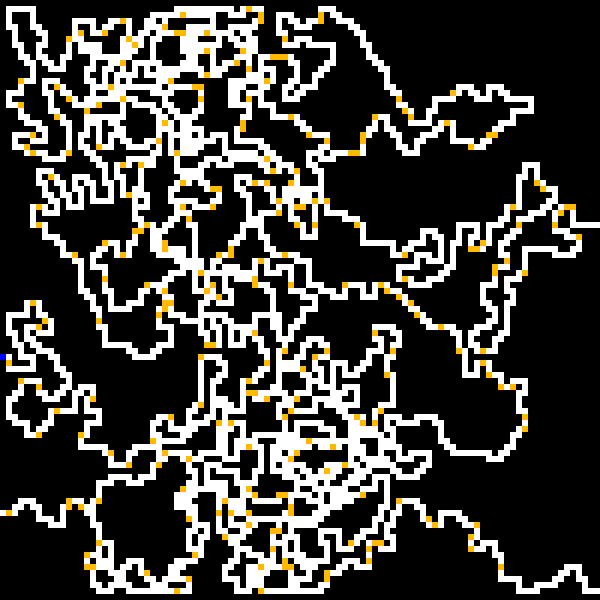1)

```seed = 2
T = 24
W = 362
H = 210
D = 15
p = 0.055303806575227585
q = 0.08780019518261352
```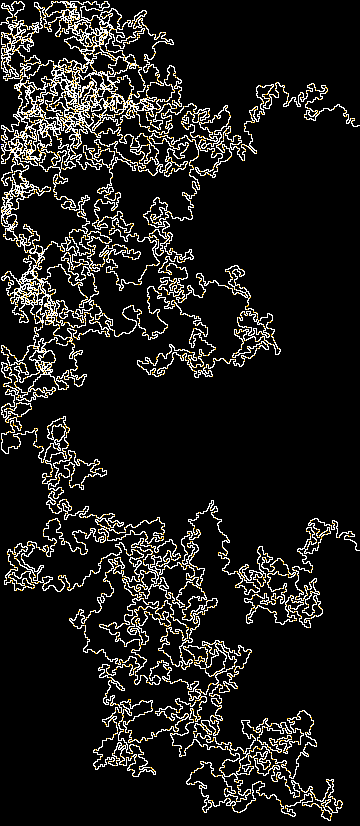2)

```seed = 3
T = 83
W = 681
H = 323
D = 18
p = 0.0548661956362973
q = 0.08317885900480129
```3)

```seed = 4
T = 79
W = 651
H = 986
D = 2
p = 0.058564166989575304
q = 0.02001374685797388
```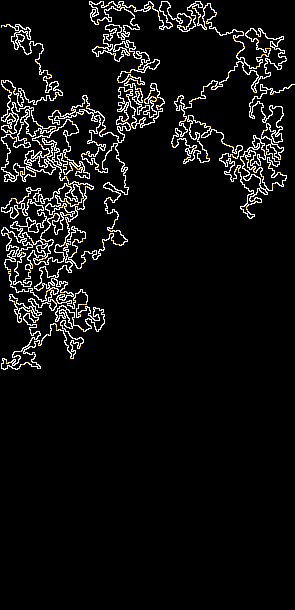4)

```seed = 5
T = 36
W = 203
H = 677
D = 17
p = 0.07970853015803138
q = 0.09490294259728929
```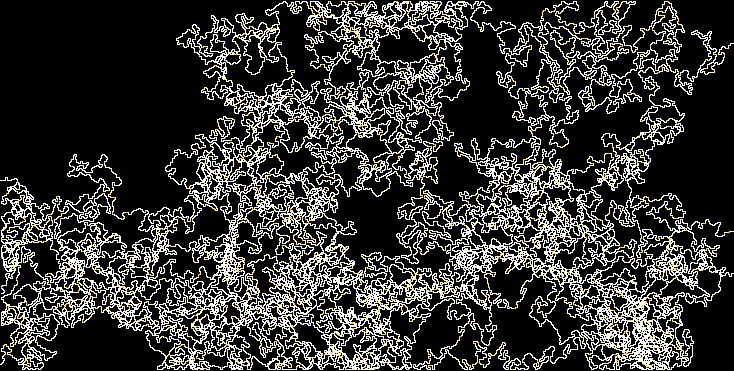5)

```seed = 6
T = 65
W = 159
H = 812
D = 5
p = 0.033369073585745014
q = 0.045186942516125796
```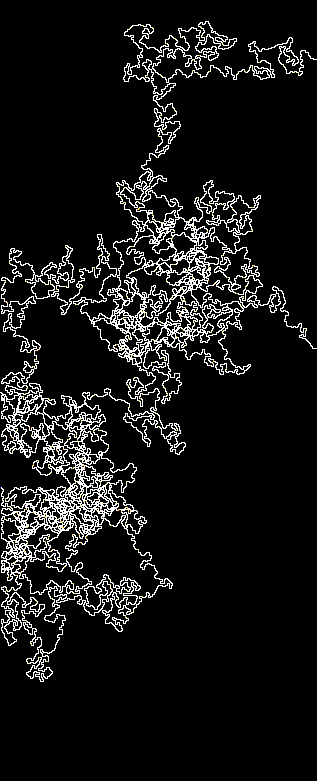6)

```seed = 7
T = 46
W = 992
H = 470
D = 6
p = 0.055533610841261474
q = 0.06802483884497222
```7)

```seed = 8
T = 69
W = 290
H = 141
D = 8
p = 0.02084588187989317
q = 0.017591207458467862
```8)

```seed = 9
T = 83
W = 352
H = 366
D = 12
p = 0.08888917594076315
q = 0.024309811436622995
```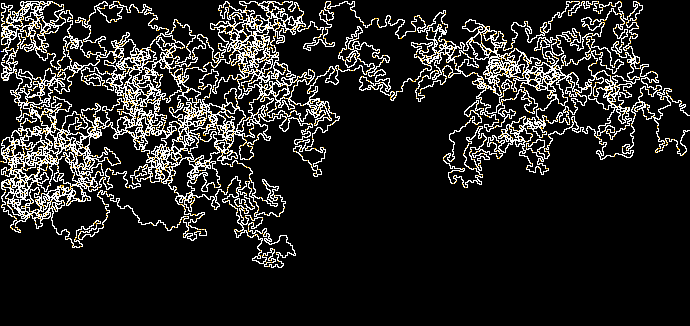9)

```seed = 10
T = 84
W = 612
H = 713
D = 1
p = 0.08528507678047445
q = 0.08388886092364066
```This problem statement is the exclusive and proprietary property of TopCoder, Inc. Any unauthorized use or reproduction of this information without the prior written consent of TopCoder, Inc. is strictly prohibited. (c)2010, TopCoder, Inc. All rights reserved.